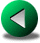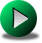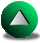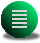Previous: The Quasi-Geostrophic Model
Next: Figures
Up: Finite Difference Methods in

# Discussion and Conclusion

Due to their fractal nature, realistic coastlines have features down to the model resolution. While the ultimate goal would be to correctly account for such features in models, a less stringent test is that models should be able to deal with simple geometries, in a manner that is not sensitive to artificial steps introduced by the discretization. Such was the study of AM, based on free-slip single gyre Munk experiments. As we noted that there was an inconsistency in the discretized vorticity budget for the C-grid shallow water model, we decided to revisit the AM results in terms of global vorticity budgets with varying resolution. Our goal was to investigate the influence of the formulation of the advective and viscous terms on the model vorticity budget and the overall strength of the gyre.

AM showed that the conventional viscous stress tensor formulation was inappropriate in the rotated basin case, for steps occurring along the coast. Moreover, they made use of an alternative stress tensor formulation (called herein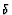-tensor) and showed improved results. We analyzed further the difference between conventional and-tensor formulation along with two different formulations of the advection in the momentum equations in term of global vorticity budgets with varying resolution. One observation is that the results with the-stress tensor depend strongly on the formulation of the advection, as the conventional advection formulation leads to instability (the D combination). Therefore, the formulation of the advection seems equally important in explaining the AM results. In terms of vorticity budgets, all combinations seem to be ill-behaved except for the enstrophy conserving advection and the-tensor (the B combination). For this combination, the convergence order for $\foa$ is about unity, following the truncation order of the vorticity when derived from second order velocity

For the QG model, the overall circulation is less sensitive to the rotation of basin for all Jacobians we tried. In order of increasing accuracy, $J_1$ gives the lowest level of accuracy (showing even signs of instability at low resolution), followed by $J_3$ and then $J_7$. The best convergence order for $\foaaa$ was obtained by using the $J_7$ Jacobian and was about 2, for all rotation angles. The beta contribution, $\fc$, is independent of the formulation of the Jacobian. Its convergence order is very close to unity and its magnitude is usually larger than that of $\foaaa$. Therefore, most of the discrepancy between the real and the model vorticity budgets is concentrated in the beta contribution at sufficiently high resolution. Hence, in order to make accurate vorticity budgets, it follows that the beta contribution should be more accurately computed. One possibility is to increase the order of the finite differencing operator for the beta term, $\beta \partial_y \psi$. Finally, the hypothesis that the $J_3$-QG model would give similar results compared to the enstrophy conserving advection and the-tensor C-grid model was verified.

From the general point of view of computing vorticity budgets from finite difference models, both QG and C-grid models show the same relatively slow convergence order (about unity) of the discrepancy with increasing resolution between the real and the model vorticity budgets. For the range of resolution considered, and depending on the model type (SW or QG), numerical formulations and the rotation angle (or more generally, basin geometry), this error can be estimated to vary between 5% to 50%.

As for the general accuracy of FD models in presence of steps for wind-driven circulations under free-slip, the rotated square basin experiments show that the B combination for the C-grid model and the $J_3$ or $J_7$ for QG model give satisfactory results. We were not able to get satisfactory results out of a conventional B-grid model, though. This spatial staggering of velocity and elevation does not seem to suit very well the free-slip boundary condition, even in presence of straight walls. The next chapter considers the more general case of a smoothly varying coastline for the Munk problem.

Frederic Dupont
2001-09-11